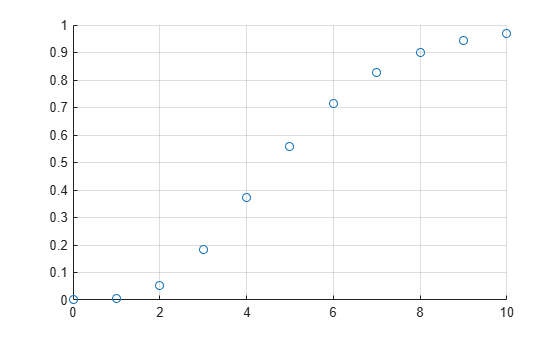# poisscdf

Poisson cumulative distribution function

## Syntax

``y = poisscdf(x,lambda)``
``y = poisscdf(x,lambda,'upper')``

## Description

example

````y = poisscdf(x,lambda)` computes the Poisson cumulative distribution function at each of the values in `x` using the rate parameters in `lambda`.`x` and `lambda` can be scalars, vectors, matrices, or multidimensional arrays that all have the same size. If only one argument is a scalar, `poisscdf` expands it to a constant array with the same dimensions as the other argument.```

example

````y = poisscdf(x,lambda,'upper')` returns the complement of the Poisson cumulative distribution function at each value in `x`, using an algorithm that computes the extreme upper tail probabilities more accurately.```

## Examples

collapse all

Compute and plot the Poisson cumulative distribution function for the specified range of integer values and average rate.

A computer hard disk manufacturing facility performs random tests of individual hard disks. The policy is to shut down the manufacturing process if an inspector finds more than four bad sectors on a disk. Assuming that on average a disk has two bad sectors, find the probability of a manufacturing process shutdown after the first inspection.

`1 - poisscdf(4,2)`
```ans = 0.0527 ```

Compute the probabilities a manufacturing process shutdown after the first inspection if on average a disk has 0, 1, 2, ..., 10 bad sectors.

```lambda = 0:10; y = 1 - poisscdf(4,lambda);```

Plot the results.

```scatter(lambda,y,'Marker',"o") grid on```Compute the complement of the Poisson cumulative distribution function with more accurate upper tail probabilities.

A computer hard disk manufacturing facility performs random tests of individual hard disks. Assuming that on average a disk has 10 bad sectors, find the probability that a disk has more than 100 bad sectors.

```format long 1 - poisscdf(100,10)```
```ans = 0 ```

This result shows that `poisscdf(100,10)` is so close to 1 (within `eps`) that subtracting it from 1 gives 0. To approximate the extreme upper tail probabilities better, compute the complement of the Poisson cumulative distribution function directly instead of computing the difference.

`poisscdf(100,10,'upper')`
```ans = 5.339405460719755e-64 ```

## Input Arguments

collapse all

Values at which to evaluate the Poisson cdf, specified as a scalar value or array of scalar values.

Example: `[0,1,3,4]`

Data Types: `single` | `double`

Rate parameters, specified as a positive value or array of positive values. The rate parameter indicates the average number of events in a given time interval.

Example: `2`

Data Types: `single` | `double`

## Output Arguments

collapse all

Poisson cdf values, returned as a scalar value or array of scalar values. Each element in `y` is the Poisson cdf value of the distribution evaluated at the corresponding element in `x`.

collapse all

### Poisson Cumulative Distribution Function

The Poisson cumulative distribution function lets you obtain the probability of an event occurring within a given time or space interval less than or equal to x times if on average the event occurs λ times within that interval.

The Poisson cumulative distribution function for the given values x and λ is

`$p=F\left(x|\lambda \right)={e}^{-\lambda }\sum _{i=0}^{floor\left(x\right)}\frac{{\lambda }^{i}}{i!}.$`

## Alternative Functionality

• `poisscdf` is a function specific to Poisson distribution. Statistics and Machine Learning Toolbox™ also offers the generic function `cdf`, which supports various probability distributions. To use `cdf`, specify the probability distribution name and its parameters. Alternatively, create a `PoissonDistribution` probability distribution object and pass the object as an input argument. Note that the distribution-specific function `poisscdf` is faster than the generic function `cdf`.

• Use the Probability Distribution Function app to create an interactive plot of the cumulative distribution function (cdf) or probability density function (pdf) for a probability distribution.

## Version History

Introduced before R2006a